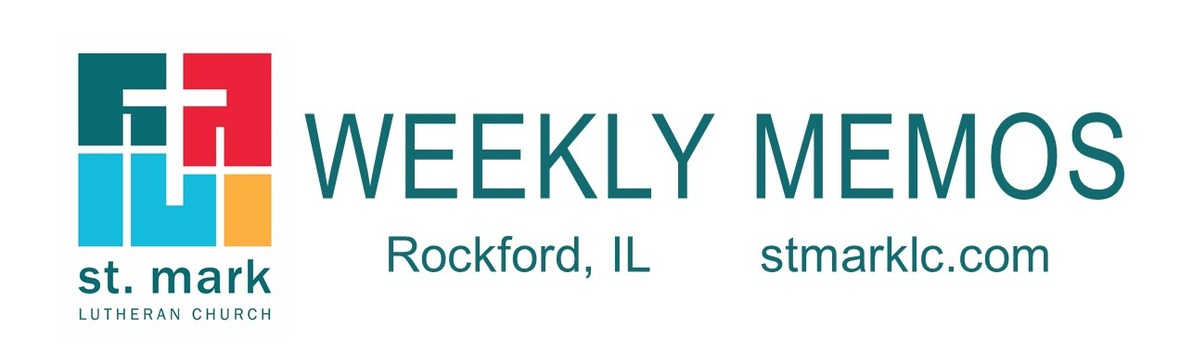table div table+table div table{width:100%;padding:0}table div table+table div table img{width:96.23%;padding:0;float:none}table div table+table div table td{width:100%;padding:0 1.88% 18px}/* styles */## This Week at St. Mark January 22-29, 2023

 table div table+table+table+table div table{width:100%;padding:0}table div table+table+table+table div table img{width:96.23%;padding:0;float:none}table div table+table+table+table div table td{width:100%;padding:0 1.88% 18px}/* styles */## Sunday, January 22

9:00 A.M. Worship with Live-stream
10:00 A.M. Fellowship Hour with coffee and donuts
10:10 A.M. Sunday School
11:00 A.M. Worship in Fellowship Hall
6:30 P.M. Youth Group at St. Mark

## Monday, January 23

5:30 P.M. Bible Study for Sunday School Teachers

## Tuesday, January 24

10:00 A.M. Staff Meeting
11:00 A.M. St. Mark Duplicate Bridge
7:00 P.M. Council Meeting

## Wednesday, January 25

9:00 A.M. Women's Bible Study
9:00 A.M. Men's Bible Study
4:30 P.M. Altar Guild
6:30 P.M. Confirmation
7:00 P.M. Voices of St. Mark

## Thursday, January 26

9:00 A.M. Cherry Valley Bridge Group
12:30 P.M. Sew and Sews
6:30 P.M. Emotions Anonymous
7:15 P.M. Red Lights

## Friday, January 27

7:30 A.M. St. Mark Breakfast Group at Denny's Restaurant
11:00 A.M. Memorial Service for Jeanette Carlson
12:00 P.M. Luncheon for Jeanette Carlson

## Saturday, January 28

10:00 A.M. Visitation for Norm Kortus
11:00 A.M. Memorial Service for Norm Kortus
12:00 P.M. Luncheon for Norm Kortus

## Sunday, January 29

9:00 A.M. Worship with Live-Stream
10:00 A.M. Fellowship Hour with coffee and donuts
10:10 A.M. Sunday Morning Volunteer Training in Sanctuary
10:10 A.M. Sunday School
11:00 A.M. Worship in Fellowship Hall

 table div table+table+table+table+table+table div table{width:100%;padding:0}table div table+table+table+table+table+table div table img{width:96.23%;padding:0;float:none}table div table+table+table+table+table+table div table td{width:100%;padding:0 1.88% 18px}/* styles */## News and Events

 table div table+table+table+table+table+table+table+table div table{width:100%;padding:0}table div table+table+table+table+table+table+table+table div table img{width:96.23%;padding:0;float:none}table div table+table+table+table+table+table+table+table div table td{width:100%;padding:0 1.88% 18px}/* styles */table div table+table+table+table+table+table+table+table+table+table div table{width:100%;padding:0}table div table+table+table+table+table+table+table+table+table+table div table img{width:96.23%;padding:0;float:none}table div table+table+table+table+table+table+table+table+table+table div table td{width:100%;padding:0 1.88% 18px}/* styles */Training for Sunday Volunteers
There will be a training session for Sunday morning volunteers on Sunday, January 29 at 10:10 in the Sanctuary during the Learning Hour. This is for both new and seasoned volunteers. This includes ushers, greeters, communion assistants, and lectors from both services. The training will be led by Pastor Chad and will last until 10:55.

 table div table+table+table+table+table+table+table+table+table+table+table+table div table{width:100%;padding:0}table div table+table+table+table+table+table+table+table+table+table+table+table div table img{width:96.23%;padding:0;float:none}table div table+table+table+table+table+table+table+table+table+table+table+table div table td{width:100%;padding:0 1.88% 18px}/* styles */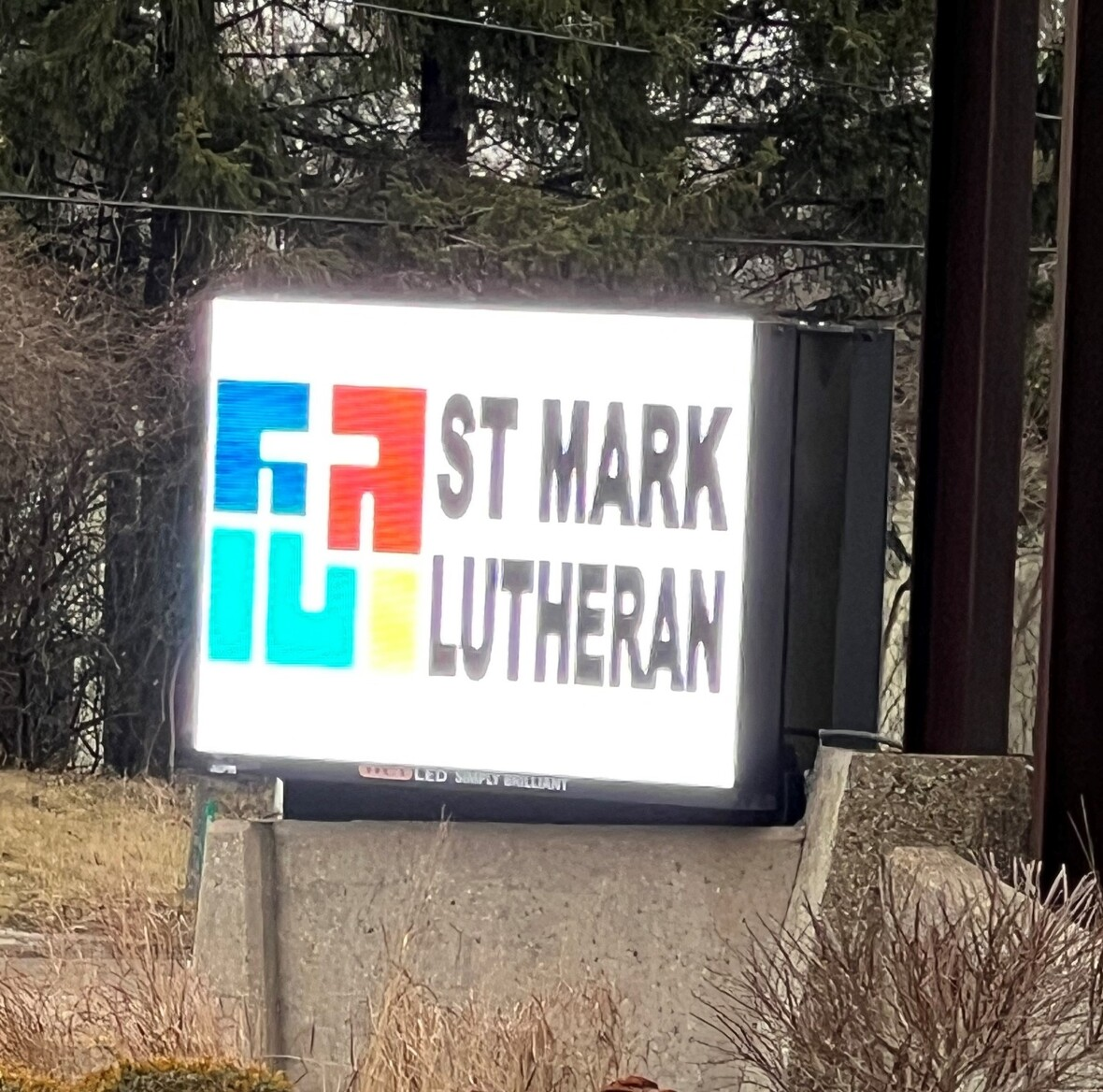Have you seen it? The new outdoor electric sign? You can't miss it! Thank you to those who donated to make this project happen.
The new sign is twice as big and will surely give travelers plenty of time to learn about some of the wonderful ministries we have going on here at St. Mark! Thank you again for your generosity. St. Mark is truly blessed.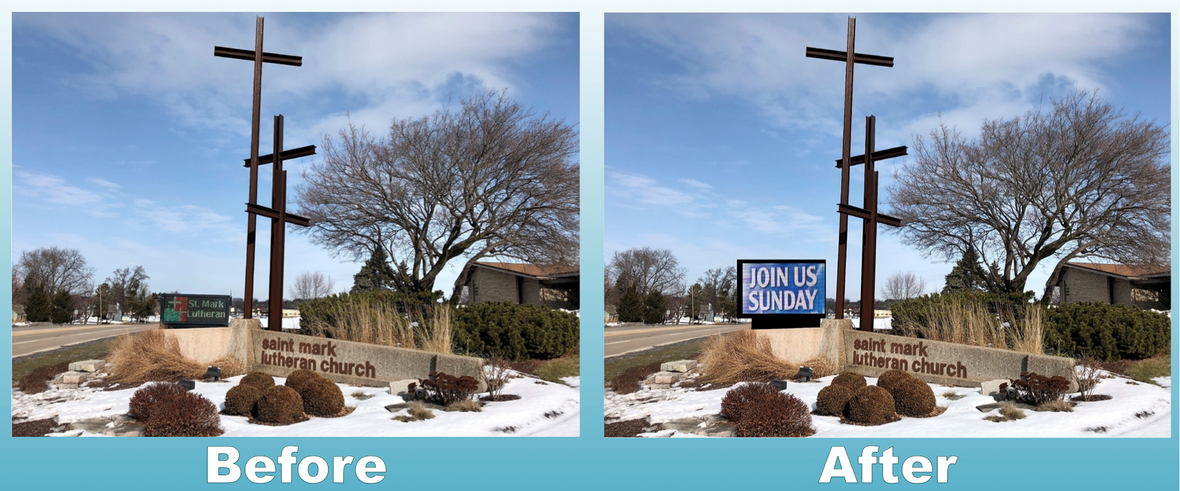table div table+table+table+table+table+table+table+table+table+table+table+table+table+table+table div table{width:100%;padding:0}table div table+table+table+table+table+table+table+table+table+table+table+table+table+table+table div table img{width:96.23%;padding:0;float:none}table div table+table+table+table+table+table+table+table+table+table+table+table+table+table+table div table td{width:100%;padding:0 1.88% 18px}/* styles */Support Groups at St. Mark
St. Mark hosts a first responder support group on Thursday nights. The 1st and 3rd Thursdays are at 7:15 pm and are for active and retired law enforcement. The 2nd and 4th Thursdays are at 7:15 pm and are for active and retired firefighters, EMS, and ED staff. All meetings will be held in the Library or Fellowship Hall, depending on group size. Contact Mark Honzel at 815-985-2101 or dmhonzel@comcast.net for more information.

 table div table+table+table+table+table+table+table+table+table+table+table+table+table+table+table+table+table div table{width:100%;padding:0}table div table+table+table+table+table+table+table+table+table+table+table+table+table+table+table+table+table div table img{width:96.23%;padding:0;float:none}table div table+table+table+table+table+table+table+table+table+table+table+table+table+table+table+table+table div table td{width:100%;padding:0 1.88% 18px}/* styles */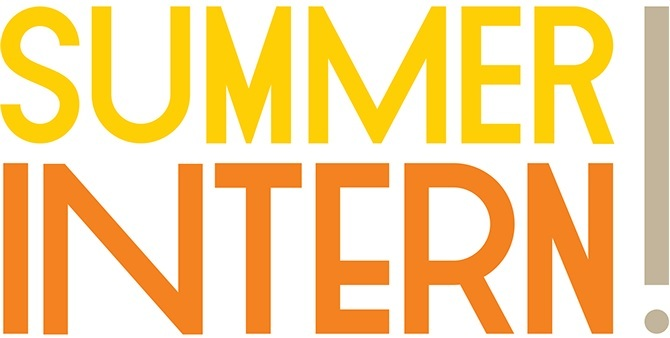Looking for a Summer Internship?
Attention College-aged members of St. Mark, the Summer Intern application for 2023 is live. Please click here at this link to fill out the application if you are interested in being the Summer Intern. If you have any questions please email Pastor
Katrina at prkatrina@stmarklc.com.

 table div table+table+table+table+table+table+table+table+table+table+table+table+table+table+table+table+table+table+table div table{width:100%;padding:0}table div table+table+table+table+table+table+table+table+table+table+table+table+table+table+table+table+table+table+table div table img{width:96.23%;padding:0;float:none}table div table+table+table+table+table+table+table+table+table+table+table+table+table+table+table+table+table+table+table div table td{width:100%;padding:0 1.88% 18px}/* styles */St Mark’s Annual Meeting
This year's annual congregation meeting is Sunday, February 12 at 10:10 a.m. It is very important as a member of St. Mark to take time to be here. There will be no 11:00 worship service this day, Sunday School or Adult Forum. The meeting will include the pastoral report on the state of the congregation, the election of officers and committee members, and a vote on amendments to our governing documents. The Fiscal 2023 budget will be presented for discussion and approval. All voting members are asked to participate. There will be plenty of fun activities for children in Fellowship Hall during the meeting.

 table div table+table+table+table+table+table+table+table+table+table+table+table+table+table+table+table+table+table+table+table+table div table{width:100%;padding:0}table div table+table+table+table+table+table+table+table+table+table+table+table+table+table+table+table+table+table+table+table+table div table img{width:96.23%;padding:0;float:none}table div table+table+table+table+table+table+table+table+table+table+table+table+table+table+table+table+table+table+table+table+table div table td{width:100%;padding:0 1.88% 18px}/* styles */A New Viereck Book
When Pastor Wayne Viereck passed away in March 2021, he left behind the unfinished manuscript of what was to have been his second and probably final book. Thanks to his wife Pat and their three daughters, work on the manuscript has been finalized and the book is now available. After finishing his first book, “Then is Now: Reading the New Testament in the 21st Century,” (2017) and the numerous studies and discussions that followed, there were many requests for Pastor Wayne to not stop there but to give us more. That is just what he has done in this new book, “Christian Faith in the 21st Century.” It is a highly readable discussion of issues ranging from the existence of God to the origin of life to Heaven and Hell and the end of the world. He concludes with a discussion of other religions, atheism, agnosticism, and secularism. The book is available on Amazon; it is \$5.99.

Pastor Wayne R. Viereck served at St. Mark from 1969-1991. He had been a parish pastor of the ELCA for 59 years. He received his undergraduate degree in English and History from the University of Wisconsin, and both his Master of Sacred Theology and Doctor of Ministry degrees from the Lutheran School of Theology.

 table div table+table+table+table+table+table+table+table+table+table+table+table+table+table+table+table+table+table+table+table+table+table+table div table{width:100%;padding:0}table div table+table+table+table+table+table+table+table+table+table+table+table+table+table+table+table+table+table+table+table+table+table+table div table img{width:96.23%;padding:0;float:none}table div table+table+table+table+table+table+table+table+table+table+table+table+table+table+table+table+table+table+table+table+table+table+table div table td{width:100%;padding:0 1.88% 18px}/* styles */The 2023 Altar Flower Chart is Up
Each Sunday we have two beautiful bouquets of flowers on the altar. The 2023 flower chart is up in the narthex on the bulletin board. You may sign up on the chart, call the church office or click on this link. We will continue to purchase them from Cherry Blossom. The price per arrangement will stay the same \$40. If you sign up online, put your memory or honor of in the comment line and pay here or write a check to St. Mark. If you have the mobile app you can do all of it on there. You may take your flowers home after the service or stop by the office
during the week to pick them up.

 table div table+table+table+table+table+table+table+table+table+table+table+table+table+table+table+table+table+table+table+table+table+table+table+table+table div table{width:100%;padding:0}table div table+table+table+table+table+table+table+table+table+table+table+table+table+table+table+table+table+table+table+table+table+table+table+table+table div table img{width:96.23%;padding:0;float:none}table div table+table+table+table+table+table+table+table+table+table+table+table+table+table+table+table+table+table+table+table+table+table+table+table+table div table td{width:100%;padding:0 1.88% 18px}/* styles */Scam Awareness
Please be vigilant when being contacted by anyone asking for money, gifts cards, etc. Always check the source, or call the church office before you reply. The church would never text you requesting an item or money. Stay safe!

 table div table+table+table+table+table+table+table+table+table+table+table+table+table+table+table+table+table+table+table+table+table+table+table+table+table+table+table div table{width:100%;padding:0}table div table+table+table+table+table+table+table+table+table+table+table+table+table+table+table+table+table+table+table+table+table+table+table+table+table+table+table div table img{width:96.23%;padding:0;float:none}table div table+table+table+table+table+table+table+table+table+table+table+table+table+table+table+table+table+table+table+table+table+table+table+table+table+table+table div table td{width:100%;padding:0 1.88% 18px}/* styles */## Outreach Ministry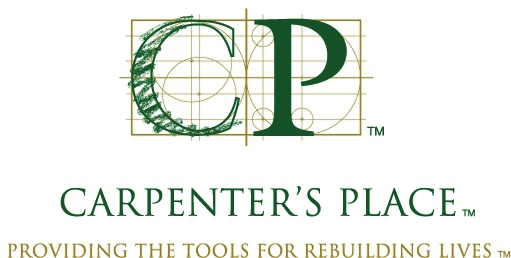Outreach Opportunity at Carpenters Place
Here is a quick and easy opportunity for St. Mark members to help serve a noon lunch at Carpenters Place this year. NO FOOD PREPARATION - Just arrive at Carpenters Place by 11:45. We serve lunch from 12:00 til 12:30. No clean-up is involved. We need 3 or 4 volunteers for the following dates in 2023:

February 23, April 27, June 22, August 24,
October 26, and December 28. Call or Text Keith @ 815-997-4499 if you are able to help.Meals for Rock House Kids
St. Mark has provided meals for the children at Rock House Kids for quite a while. The next time we are scheduled to provide a meal is Wednesday, February 15. Wednesdays are one of Rock House Kids busiest nights, they get approximately 120 kids. What we have done in the past is order the food from Gray’s on Alpine either Tater-tot Casserole or Spaghetti Casserole, both are very good. We also provide a veggie (like carrots and ranch), bread and cookies, which can be purchased at Sam’s or Costco. The meal is picked up around 4:30ish from Gray’s and dropped off at Rock House between 5 and 5:30. It is pretty easy if you order the main dish. If the cost of providing food for 120 kids is concerning, there is money left on a Thrivent Action Grant from the last time we provided a meal which should cover most of the cost for the February meal. If you have any questions please reach out to Pastor Katrina. If you are interested in helping provide a meal for Rock House Kids, either for February or future meals (St. Mark typically provides a meal once a month or once every other month), please let Pastor Katrina or Jill Davenport know. Thank you!

 table div table+table+table+table+table+table+table+table+table+table+table+table+table+table+table+table+table+table+table+table+table+table+table+table+table+table+table+table+table+table+table div table{width:100%;padding:0}table div table+table+table+table+table+table+table+table+table+table+table+table+table+table+table+table+table+table+table+table+table+table+table+table+table+table+table+table+table+table+table div table img{width:96.23%;padding:0;float:none}table div table+table+table+table+table+table+table+table+table+table+table+table+table+table+table+table+table+table+table+table+table+table+table+table+table+table+table+table+table+table+table div table td{width:100%;padding:0 1.88% 18px}/* styles */## Youth MinistrySunday Youth Group Schedule for January
St. Mark Youth Group will meet at St. Mark on Sunday, January 22 at 6:30 pm.

 /* styles */
 table div table+table+table+table+table+table+table+table+table+table+table+table+table+table+table+table+table+table+table+table+table+table+table+table+table+table+table+table+table+table+table+table+table+table+table div table{width:100%;padding:0}table div table+table+table+table+table+table+table+table+table+table+table+table+table+table+table+table+table+table+table+table+table+table+table+table+table+table+table+table+table+table+table+table+table+table+table div table img{width:96.23%;padding:0;float:none}table div table+table+table+table+table+table+table+table+table+table+table+table+table+table+table+table+table+table+table+table+table+table+table+table+table+table+table+table+table+table+table+table+table+table+table div table td{width:100%;padding:0 1.88% 18px}/* styles */table div table+table+table+table+table+table+table+table+table+table+table+table+table+table+table+table+table+table+table+table+table+table+table+table+table+table+table+table+table+table+table+table+table+table+table+table+table div table{width:100%;padding:0}table div table+table+table+table+table+table+table+table+table+table+table+table+table+table+table+table+table+table+table+table+table+table+table+table+table+table+table+table+table+table+table+table+table+table+table+table+table div table img{width:96.23%;padding:0;float:none}table div table+table+table+table+table+table+table+table+table+table+table+table+table+table+table+table+table+table+table+table+table+table+table+table+table+table+table+table+table+table+table+table+table+table+table+table+table div table td{width:100%;padding:0 1.88% 18px}/* styles */## Remember In Our Prayers

 table div table+table+table+table+table+table+table+table+table+table+table+table+table+table+table+table+table+table+table+table+table+table+table+table+table+table+table+table+table+table+table+table+table+table+table+table+table+table+table div table{width:100%;padding:0}table div table+table+table+table+table+table+table+table+table+table+table+table+table+table+table+table+table+table+table+table+table+table+table+table+table+table+table+table+table+table+table+table+table+table+table+table+table+table+table div table img{width:96.23%;padding:0;float:none}table div table+table+table+table+table+table+table+table+table+table+table+table+table+table+table+table+table+table+table+table+table+table+table+table+table+table+table+table+table+table+table+table+table+table+table+table+table+table+table div table td{width:100%;padding:0 1.88% 18px}/* styles */## Our St. Mark members:

Lisa Belk, Lorine Kortus, Beth Johnson, Carol Kilts, Tim Panjkovich, Rita Olson, Fritzi Panjkovich, Bev Johnson, Jack Sowl, Jan Cotter, Diane Swanson, Sherri Lindquist, Bette Patterson.

## Our condolences to:

Jerry Heinzeroth and family upon the death of his wife, Barbara Heinzeroth and to Craig and Janet Bederka upon the death of Janet’s mother, Margit Hohl.

## Family and friends of St. Mark members:

Denise Nichols, Carrie Nack, Larry Foster, Barbara, Buttchen, John Ayotte, Carrie Kielty, Ed Sobczyk, Jeff Basset, Elizabeth Woodworth, Carrie Nelson, Bill Hart, Ellie Krepfle, Don Minnis, Tom Montgomery, Jon Baylander, John Mortensen, Carolyn Neagle, Jenny Hobby, Bill King, Carolyn King, Norma Comstock.

## Those serving in the military and their families:

Logan Gehrke, Hunter Haggestad, Tristan Davenport, Scot Hornick,
Bailey Panjkovich, Jim Tammen, Brandon Ballenger, Taylor Eversole.

Strengthen and encourage those in public health services and in the medical profession: care-givers, nurses, attendants, doctors, all who commit themselves to care for the sick and their families.

 table div table+table+table+table+table+table+table+table+table+table+table+table+table+table+table+table+table+table+table+table+table+table+table+table+table+table+table+table+table+table+table+table+table+table+table+table+table+table+table+table+table div table{width:100%;padding:0}table div table+table+table+table+table+table+table+table+table+table+table+table+table+table+table+table+table+table+table+table+table+table+table+table+table+table+table+table+table+table+table+table+table+table+table+table+table+table+table+table+table div table img{width:96.23%;padding:0;float:none}table div table+table+table+table+table+table+table+table+table+table+table+table+table+table+table+table+table+table+table+table+table+table+table+table+table+table+table+table+table+table+table+table+table+table+table+table+table+table+table+table+table div table td{width:100%;padding:0 1.88% 18px}/* styles *//* styles */ Office Hours Monday through Thursday 9:00 A.M. - 4:00 P.M. Friday - 9:00 A.M. - 1:00 P.M. Telephone: 815-398-3557 Publications St. Mark Memos, the weekly e-newsletter, is distributed each Thursday. The deadline for submission is Monday by 3:00 P.M. Please click to send submissions. Worship Services Miss a Sunday at St. Mark? Please visit our website and watch the service online. Please click to watch.
 table div table+table+table+table+table+table+table+table+table+table+table+table+table+table+table+table+table+table+table+table+table+table+table+table+table+table+table+table+table+table+table+table+table+table+table+table+table+table+table+table+table+table+table div table{width:100%;padding:0}table div table+table+table+table+table+table+table+table+table+table+table+table+table+table+table+table+table+table+table+table+table+table+table+table+table+table+table+table+table+table+table+table+table+table+table+table+table+table+table+table+table+table+table div table img{width:96.23%;padding:0;float:none}table div table+table+table+table+table+table+table+table+table+table+table+table+table+table+table+table+table+table+table+table+table+table+table+table+table+table+table+table+table+table+table+table+table+table+table+table+table+table+table+table+table+table+table div table td{width:100%;padding:0 1.88% 18px}/* styles */